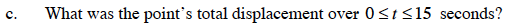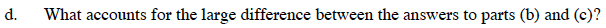### Home > CALC > Chapter 9 > Lesson 9.2.2 > Problem9-69

9-69.
1. The velocity of a point moving along the x-axis is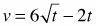units per second. Homework Help ✎

1. What was the average velocity over 0 ≤ t ≤ 15 seconds?

2. During the first 15 seconds, what is the total distance the point traveled?

3. What was the point's total displacement over 0 ≤ t ≤ 15 seconds?

4. What accounts for the large difference between the answers to parts (b) and (c)?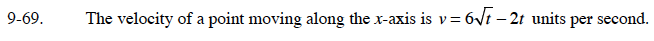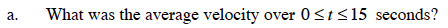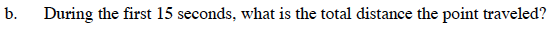Graph the velocity function to see wether or not the point changes direction in the given interval. If it does, the integral needs to be broken into parts.